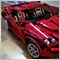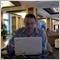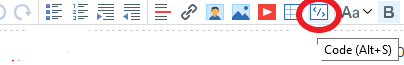# EMA Cross Over10

Hi Have created and EA with EMA crossover, but dont know what i missed from whiich i could auto buy or sell in market.

Below are the codes,

WIll be great help if any one can help me fixing it.

void OnTick()

{

// Current chart, current period, 50 candles, no shift, expotential, close price

double SlowMovingAverage=iMA(NULL,0,50,0,MODE_EMA,PRICE_CLOSE,0);

// Current chart, current period, 50 candles, no shift, expotential, close price

double LastSlowMovingAverage = iMA(NULL,0,50,0,MODE_EMA,PRICE_CLOSE,1);

// Current chart, current period, 14 candles, no shift, expotential, close price

double FastMovingAverage = iMA(NULL,0,14,0,MODE_EMA,PRICE_OPEN,0);

// Current chart, current period, 14 candles, no shift, expotential, close price

double LastFastMovingAverage = iMA(NULL,0,14,0,MODE_EMA,PRICE_OPEN,1);

// if the fast SMA is now above

if((LastFastMovingAverage < LastSlowMovingAverage)

&&(FastMovingAverage > SlowMovingAverage))

{

// if we have no open orders

if(OrdersTotal()<1)

{

// we send a buy order

// we count all the orders,

for(int b= OrdersTotal()-1; b>=0; b--)

{

// we check if the order belongs to the current chart

if(OrderSymbol()==Symbol())

{

// if it is a buy order

{

// if the stop loss is below 250 points

if (OrderStopLoss() < Ask - (250*_Point))

{

// we  modify the stop loss

OrderModify(

OrderTicket(),             // for current order

OrderOpenPrice(),          // opened for the OpenPrice

Ask - (250*_Point),        // set stop loss

OrderTakeProfit(),         // unchanged the profit

0,                         // no expiration

clrNONE);                   // no color

}

}

}

}

}

}

// if the fast SMA is now below

if((LastFastMovingAverage > LastSlowMovingAverage)

&&(FastMovingAverage < SlowMovingAverage))

{

Comment("SELL");

// if we have no open orders

if(OrdersTotal()<1)

{

// we send a sell order

int sellticket = OrderSend(Symbol(),OP_SELL,0.10,Bid,3,0,0,NULL,0,0,Red);

// we count all the orders,

for(int c= OrdersTotal()-1; c>=0; c--)

{

// we check if the order belongs to the current chart

if (OrderSymbol()==Symbol())

{

// if it is a sell order

if (OrderType()==OP_SELL)

{

// if the stop loss is 0 or above 250 points

if(OrderStopLoss()==0|| OrderStopLoss() > Bid + (250*_Point))

// we  modify the stop loss

OrderModify(

OrderTicket(),             // for current order

OrderOpenPrice(),          // opened for the OpenPrice

Bid + (250*_Point),        // set stop loss

OrderTakeProfit(),         // unchanged the profit

0,                         // no expiration

clrNONE);                   // no color

}

}

}

}

}

}1218

wonderfinance:

Hi Have created and EA with EMA crossover, but dont know what i missed from whiich i could auto buy or sell in market.

Below are the codes,

WIll be great help if any one can help me fixing it.

Can this code compile and run?

What do you see when you run it?Moderator
114388

wonderfinance:

Hi Have created and EA with EMA crossover, but dont know what i missed from whiich i could auto buy or sell in market.

Below are the codes,

...